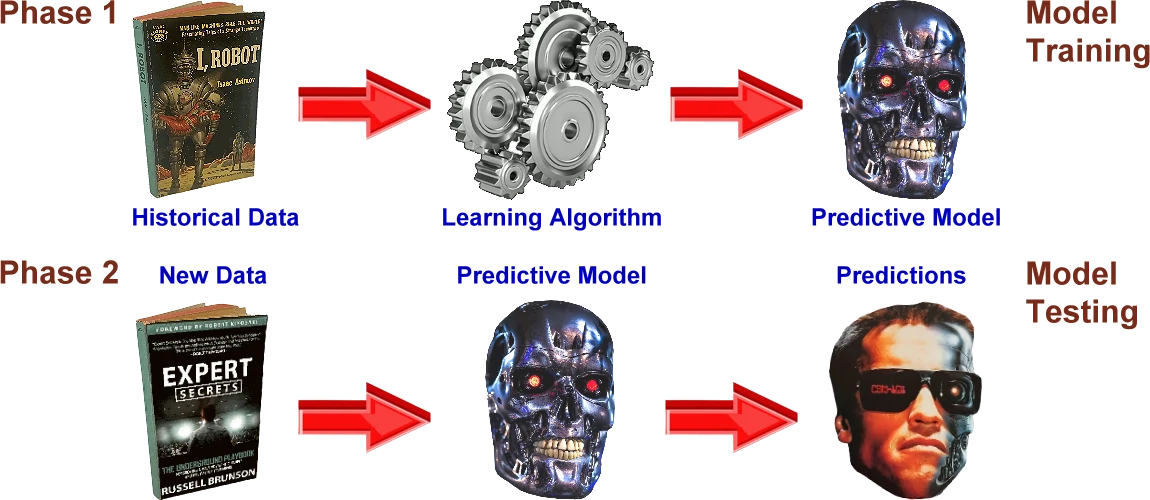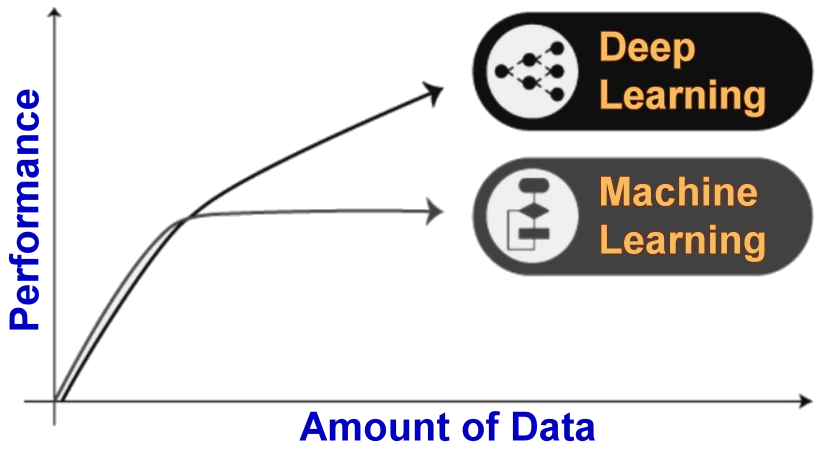# 7.12.1. Algorithms and models of AIRecently, there was an article in a some magazine about the car 'Tesla'. On some fast road, there was a collision between two cars in front of the Tesla car. The first car continued to drive, but the second car's engine was damaged and the car immediately stopped and crossed the road. The driver of the 'Tesla' did not manage immediately, and the collision avoidance algorithm in the 'Tesla' took control of the car's movement and went around the obstructed car. Namely, the average driver needs about 0.75 seconds to react to the observed situation (the F1 driver needs about 0.15 seconds), but the computer system in 'Tesla' reacts much faster through the existing sensors and applied its algorithm when it found that there was no reaction from the driver. In this way, another collision was avoided. The driver of the 'Tesla' could have fallen asleep or he could have felt ill, but 'Tesla' makes sure that no one gets hurt. Nice! So, it really benefits from artificial intelligence.

The relationship between algorithms and models in artificial intelligence is that algorithms are the computational procedures or techniques used to train or implement models. Algorithms provide the step-by-step instructions for processing data, making decisions, and learning from examples. They define the logic and rules that govern how a model learns, predicts, or performs a specific task. Different algorithms can be applied to train or optimize models based on the specific problem and the available data.

On the other hand, models represent the learned or implemented representations of knowledge or behavior. They are the result of applying algorithms to training data, and they capture the patterns, relationships, or characteristics of the data that are relevant to the specific task. Models can be considered as the operational implementations of the algorithms, which can make predictions, classify inputs, generate outputs, or perform other tasks based on the learned knowledge.

In some cases, the terms 'algorithm' and 'model' may be used interchangeably, depending on the context. However, it is useful to distinguish between them to understand the relationship. Algorithms are the underlying computational techniques, while models are the specific instantiations or implementations of those algorithms, trained or optimized for a particular task or problem. For example, in the context of neural networks, the algorithm refers to the learning algorithm such as backpropagation or stochastic gradient descent (SGD), which defines how the network adjusts its weights during training. The model, on the other hand, refers to the specific architecture and parameters of the neural network, which are determined by the training process and define how the network makes predictions or performs tasks.

Artificial intelligence (AI) algorithms are the computational procedures or mathematical formulas used to enable machines to mimic or simulate intelligent behavior. There are several basic artificial intelligence (AI) algorithms that are commonly used in a variety of applications. Here are a few examples:

• 1. Linear Regression: Linear regression is a supervised learning algorithm used for predicting continuous numerical values. It finds the best linear relationship between input features and the target variable by minimizing the sum of the squared differences between the predicted and actual values.

• 2. Logistic Regression: Logistic regression is a classification algorithm used for predicting binary or categorical outcomes. It calculates the probability of an event occurring based on input features and applies a threshold to make predictions.

• 3. Reinforcement Learning Algorithms: These algorithms learn through interaction with an environment. They receive feedback in the form of rewards or penalties and use it to improve their decision-making. Examples include Q-learning and deep reinforcement learning algorithms like Deep Q-Network (DQN).

• 4. Decision Trees: Decision trees are versatile algorithms used for both classification and regression tasks. They create a tree-like model of decisions and their possible consequences based on features, enabling the algorithm to make predictions by traversing the tree.

• 5. Random Forests: Random forests are an ensemble learning method that combines multiple decision trees. Each tree in the forest is built on a random subset of the training data, and predictions are made by aggregating the results from all the trees.

• 6. Support Vector Machines (SVM): SVM is a powerful algorithm for both classification and regression tasks. It finds a hyperplane in a high-dimensional space that separates the data into different classes, maximizing the margin between the classes.

These are just a few examples of AI algorithms, and the field is continuously evolving with new algorithms and techniques being developed to tackle various challenges in artificial intelligence. The speed of an algorithm can depend on various factors, such as the problem it is solving, the size of the input data, the hardware on which it is executed, and the specific implementation details. Additionally, algorithmic efficiency is not the only factor to consider in real-world scenarios. Other factors like memory usage, scalability, and ease of implementation can also play a role in determining the best algorithm for a given situation.

In the context of artificial intelligence, models refer to specific implementations or architectures that learn from data to perform tasks such as prediction, classification, or generation. These models can be trained on various algorithms and techniques. Models of AI that have been developed over the years. Here are a few of the most commonly used models:

• 1. Expert Systems: Expert systems are designed to mimic the decision-making ability of a human expert in a specific domain. They use a knowledge base of rules and facts and an inference engine to provide solutions or make decisions based on user input.

• 2. Neural Networks: Neural networks are computational models inspired by the structure and function of the human brain. They consist of interconnected nodes, or artificial neurons, organized in layers. Neural networks can be trained using algorithms such as backpropagation to learn patterns and make predictions.

• 3. Genetic Algorithms: Genetic algorithms are optimization algorithms based on the principles of natural selection and genetics. They involve maintaining a population of candidate solutions and using selection, mutation, and crossover operations to evolve and improve these solutions over generations.

• 4. Reinforcement Learning: Reinforcement learning is an approach to AI that involves an agent learning from interactions with an environment. The agent takes actions, receives feedback in the form of rewards or penalties, and adjusts its behavior to maximize long-term rewards. It uses techniques like Markov decision processes and Q-learning to optimize its actions.

• 5. Bayesian Networks: Bayesian networks are probabilistic graphical models that represent and reason about uncertain knowledge using Bayesian inference. They use directed acyclic graphs to model the dependencies between variables and make predictions or perform reasoning based on probabilistic calculations.

• 6. Hidden Markov Models: Hidden Markov Models (HMM) are statistical models that are used to model sequential data. They consist of a set of hidden states and observable outputs, with transitions between states governed by probabilities. HMMs are commonly used for tasks such as speech recognition and natural language processing.

These are just a few examples of basic models of AI. Each model has its own strengths, limitations, and areas of application. AI research continues to explore and develop new models to tackle complex problems and improve the capabilities of AI systems.

In summary, algorithms provide the computational techniques and procedures used to train or implement models, while models represent the learned or implemented knowledge or behavior that enables machines to perform specific tasks. A general structure of a AI learning based predictive model considering both the training and testing phase, as shown in the next Figure.Figure 7.12.4. General structure of a AI learning.

There are two main types of learning at AI system: Machine learning and Deep learning. Next Figure shows a general performance of deep learning over machine learning considering the increasing amount of data. However, it may vary depending on the data characteristics and experimental set up.Figure 7.12.5. Machine learning and deep learning performance.

Machine learning and deep learning are both subfields of artificial intelligence (AI) that involve training algorithms to learn from data and make predictions or decisions. While they are related, there are some key differences between the two.

Machine learning is a broader field that focuses on algorithms and techniques to enable computers to learn from data and make predictions or decisions. It encompasses various approaches like supervised, unsupervised, and reinforcement learning. Supervised learning is typically the task of machine learning to learn a function that maps an input to an output based on sample input-output pairs. Unsupervised learning analyzes unlabeled datasets without the need for human interference, i.e., a data-driven process. Reinforcement learning is a type of machine learning algorithm that enables software agents and machines to automatically evaluate the optimal behavior in a particular context or environment to improve its efficiency, i.e., an environment-driven approach.

Deep learning is a subset of machine learning that uses artificial neural networks inspired by the human brain. It excels at learning complex patterns and relationships in data by automatically extracting hierarchical representations. It has achieved remarkable success in areas like computer vision and natural language processing.

In short, machine learning is a general term, while deep learning is a specific approach within machine learning that leverages neural networks for complex tasks.

SUMMARY:

Is there a fear of the development of artificial intelligence or not?

• NO - If artificial intelligence adheres to the aforementioned laws of robotics described in the introductory chapter, there are no problems.

• YES - If the achievements of artificial intelligence are used for weapons of war for the purpose of eliminating enemy manpower, then we are screwed.

Who can forbid the army or limit them in what they do? Nobody! Try to imagine what 'military experts' are able to do with an aircraft of the type shown in Figure 9.1. Using artificial intelligence for military purposes is the same as building intercontinental missiles with nuclear warheads. Then the military leaders will 'agree' on limiting armaments at some summits?

What can be done, since time immemorial it has been in the nature of man to kill and make war. Why should it be different now?Citing of this page: Radic, Drago. " IT - Informatics Alphabet " Split-Croatia. {Date of access}. . Copyright © by Drago Radic. All rights reserved. | Disclaimer